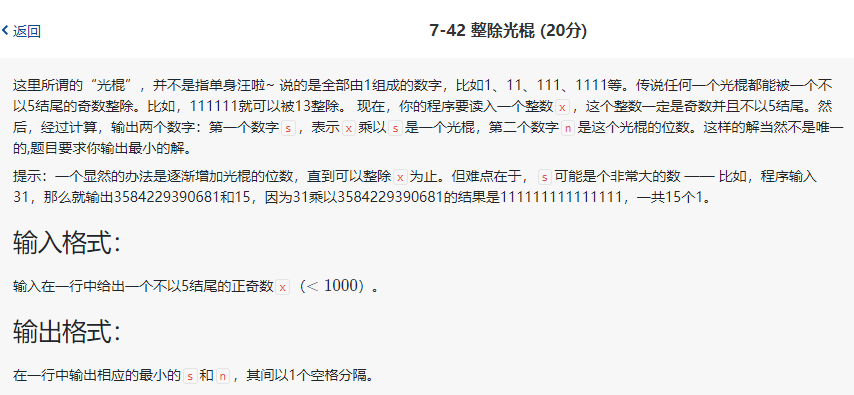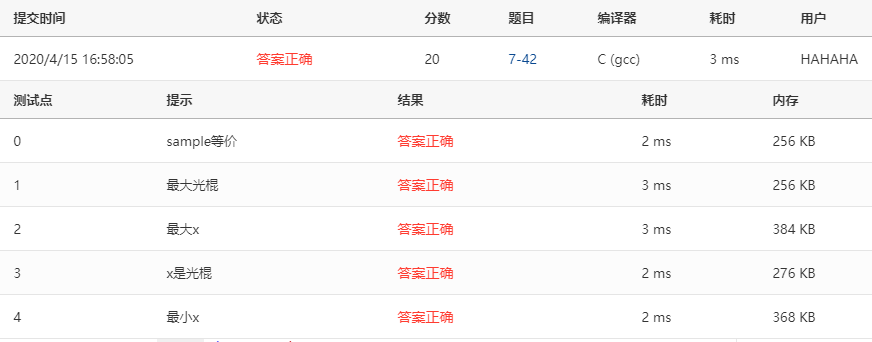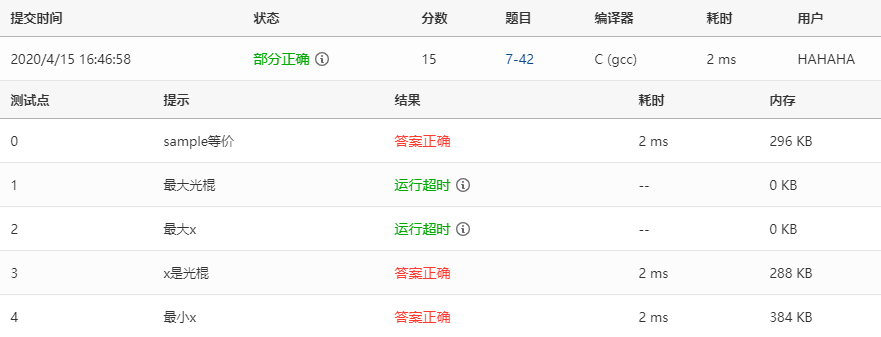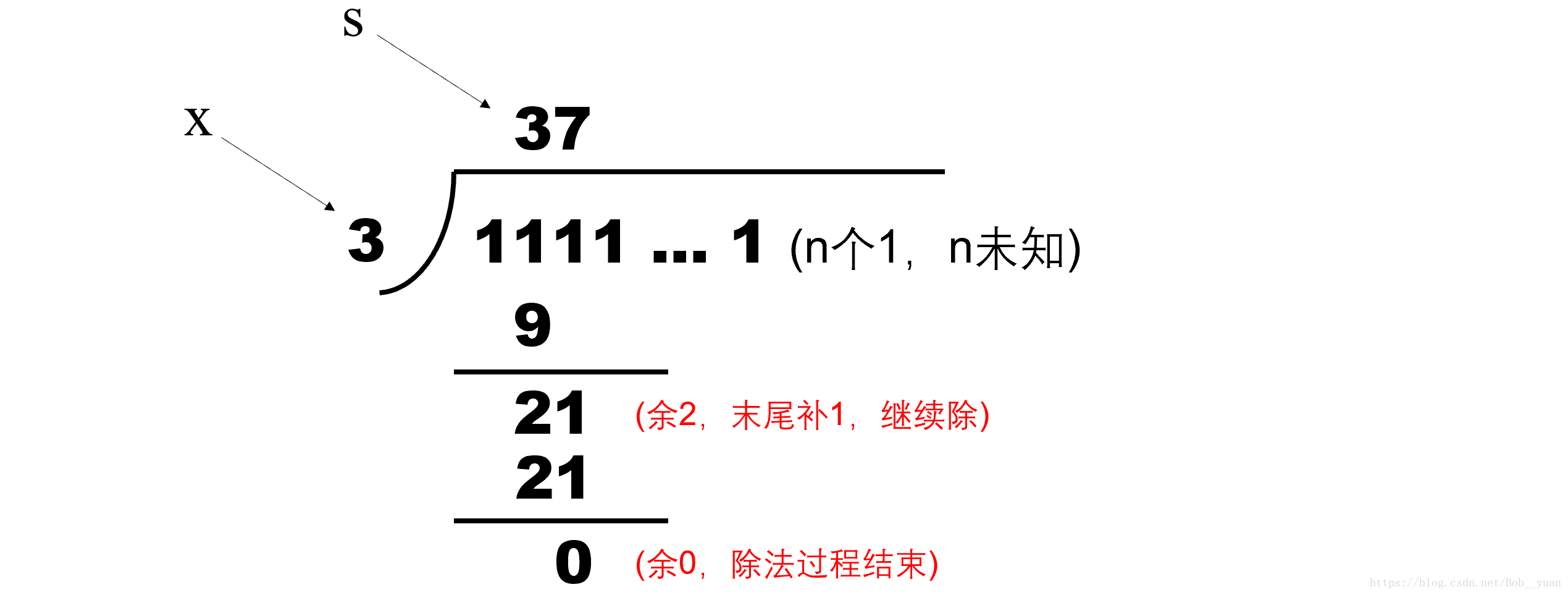# PTA刷题笔记(C语言) | 7-42 整除光棍 (20分)# 1、题目31

3584229390681 15

# 2、代码

#include<stdio.h>
int main(){
int x;
scanf("%d",&x);
int cnt=0;
int a=0,c=0,d;
while(a<x){
a=a*10+1;
cnt++;
}
while(1){
printf("%d",a/x);
a%=x;
if(a==0) break;
a=a*10+1;
cnt++;
}
printf(" %d",cnt);

return 0;
}# 3、讨论

#include<stdio.h>
#include<math.h>
int main(){
int x;
scanf("%d",&x);
int i;
int cnt=0;
long long int a=0,c=0,d;
while(1){
a=a*10+1;
cnt++;
c=a/x;
d=a%x;
if(d==0){
printf("%lld %d",c,cnt);
break;
}
}
//	printf("%lld %d",c,cnt);

return 0;
}05-155763
04-16430705-123995
11-24147
04-0484
05-08757
02-29122
03-29234
04-29375